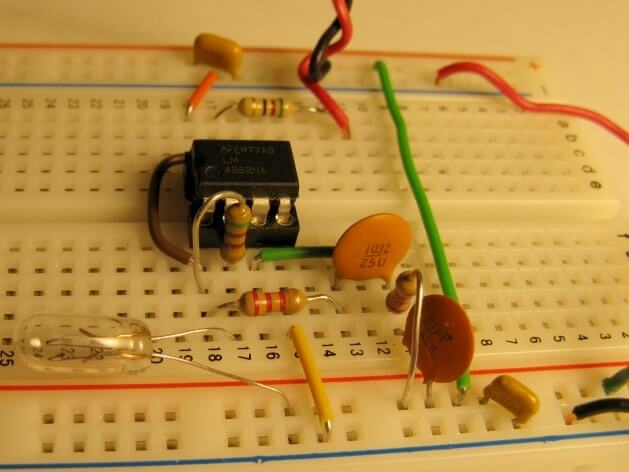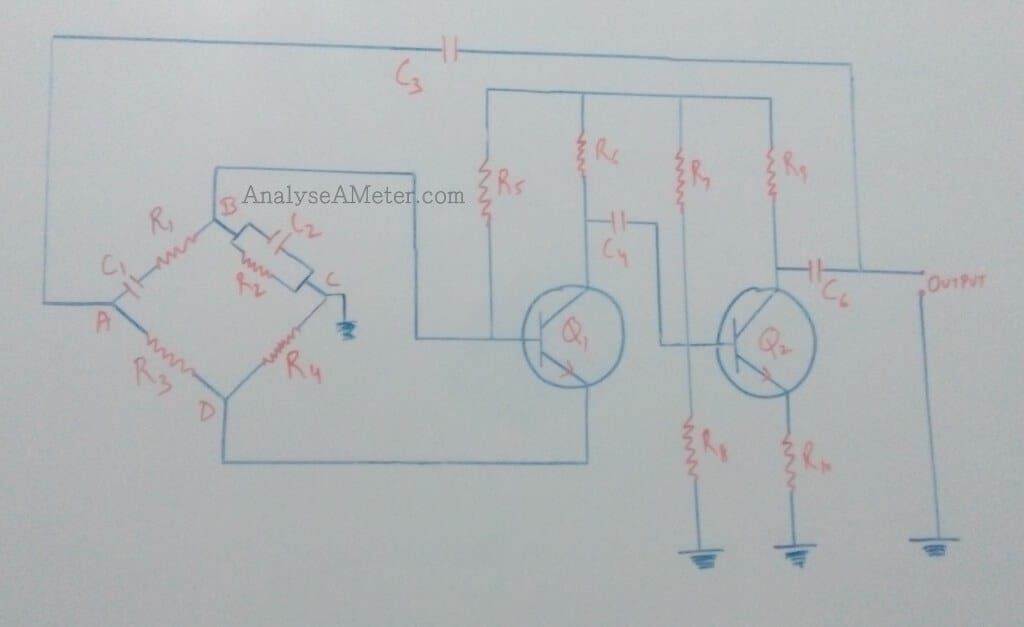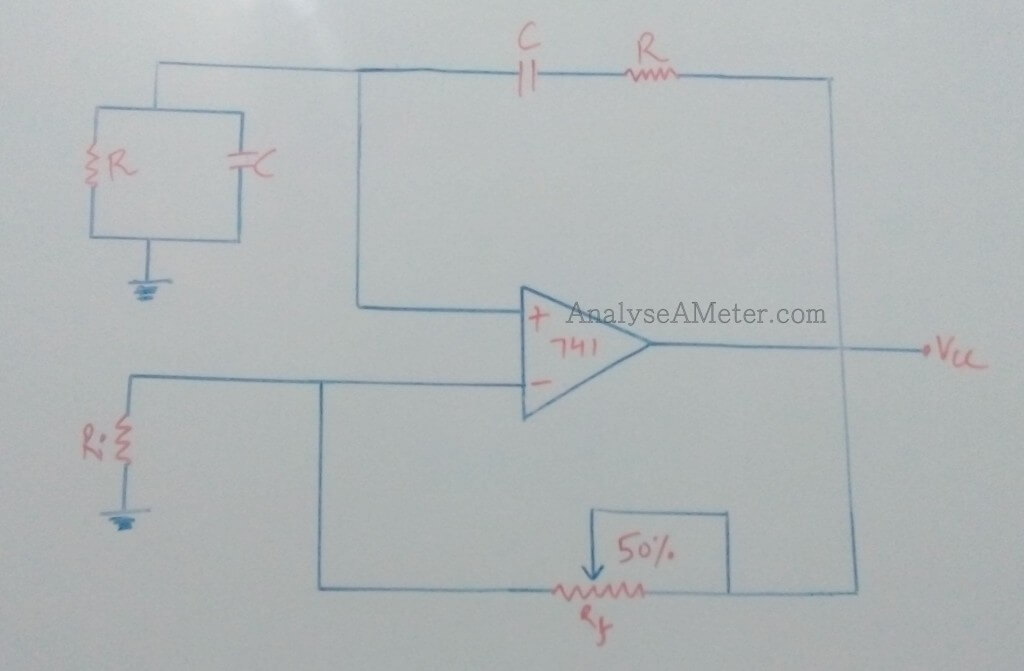# Wien bridge Oscillator Circuit and Design using Op amp

A Wien bridge oscillator is based on bridge circuit basically used to generate sine waves at a large range of frequencies. It is a two stage RC coupled amplifier circuit that has low distortion and good stability at its resonant frequency. In our previous articles, we explain LC oscillators i.e. Hartley and Colpitts oscillator and crystal oscillator in detail. Here we are going to explain Wien Bridge oscillator and its circuit in detail.For the measurement of impedances, it was developed by Max Wien in 1891. It is one of the most demandable Oscillator use in audio or sub-audio frequency ranging from 20-20KHz.

# Wien bridge oscillator circuit:Wien bridge oscillator circuit

As shown in its circuit diagram above, it is essentially a two-stage amplifier with an RC bridge circuit. In this bridge circuit, R1 is connected in series to C1 and R2, R3, R4 are connected in parallel to C2 form the four arms. In this arrangement transistor, Q1 serves as an oscillator and amplifier while transistor Q2 act as an inverter to cause a phase shift of 180o. Resistors R3 and R4 are used to stabilize the amplitude of the output. At the input of the transistor Q1, the negative feedback is applied through the voltage divider and positive feedback is through R1, C1, R2 and C2. In this circuit, Wien bridge feedback network is added to make the oscillator sensitive to a signal of only one particular frequency. If this feedback network is not employed than for producing oscillations the output of transistor Q2 is fed back to transistor Q1 for providing regeneration. The transistor Q1 will amplify signals over a wide range of frequencies and thus direct coupling would result in poor frequency stability. Thus by employing wien bridge feedback network frequency stability is increased.

It comprises of Feedback circuit consisting of a series RC circuit connected with a parallel RC of the same component value producing a phase delay depending upon the frequency. It employs two transistors each producing a phase shift of 180o, cause a total phase shift of 360 o or 0 o and ensures proper positive feedback. To ensure a constant output over a range of frequencies, a negative feedback is provided in the circuit. This can be achieved by taking resistor R4 in the form of a temperature sensitive lamp whose resistance increase with the increase in current.

As shown in the figure, the amplifier voltage gain is given as:

A = (R3 + R4) / R4 = 3

= (R3 / R4) + 1  = 3

= R3 / R4 = 2

Since R3 = 2 R4 corresponds with a feedback network attenuation of 1/3 indicates that the voltage gain of the amplifier circuit must be equal to or greater than three for oscillations to start.

## Wien Bridge oscillator using op-amp:

As shown in Figure below, the Wien bridge circuit consists of a series RC network in one arm and parallel RC network in the adjoining arm, Ri & Rf are connected in the remaining arm. The op-amp used in this oscillator circuit is working as non-inverting amplifier mode. Components required for this circuit are a capacitor, potentiometer, resistor and an operational amplifier.Wien bridge circuit diagram using op-amp

In this oscillator circuit, the feedback signal is connected to the non-inverting input terminal so that the op-amp works as a non-inverting amplifier. For sustained oscillations, zero phase shift is essential across the circuit which is achieved by balancing the bridge. At resonance frequency, the inverting and non-inverting voltages will be equal and in phase so that the negative feedback signal will be canceled out by the positive feedback causing the circuit to oscillate. The resonant frequency of the balanced bridge represents the frequency of oscillations given as:

fo = 1 / 2∏ RC

For an inverting amplifier, the gain is set by the feedback resistor network Ri & Rf and is given by the ratio of -Rf / Ri  and also represents that for sustained oscillations amplifier must have a gain of 3 so that the loop gain become unity.

### Advantages and Disadvantages of Wien bridge oscillator:

Wien bridge oscillator is an electronic oscillator used in many applications. Following of its advantages are:

• By using decade resistance boxes, the frequency range can be selected easily.
• Over a wide range of frequency, it provides a stable low distortion sinusoidal output.
• By varying capacitances C1 and C2 simultaneously, the frequency of oscillation can be easily varied.

Like a coin has two sides, similarly, there are some problems occur while using these type of oscillators. Following of disadvantages are:

• The maximum frequency output is limited because of amplitude and phase shift characteristics of the amplifier.
• The design is bulky because circuit requires two transistors and a large number of other electronic components.

Hope you all like this article. For any suggestions please comment below. We always appreciate your suggestions.

Like this? Vote now
[Total: 1 Average: 5]

### 1 thought on “Wien bridge Oscillator Circuit and Design using Op amp”

This site uses Akismet to reduce spam. Learn how your comment data is processed.

error: Stop Stealing content, do some hardwork !!